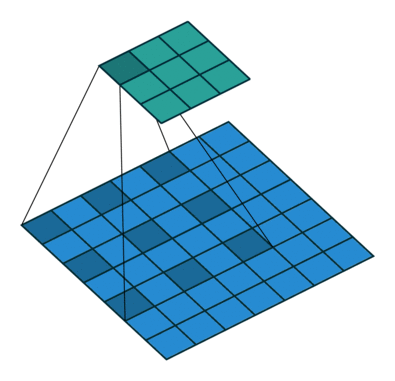# SWAP: Softmax-Weighted Average PoolingWe present a pooling method for convolutional neural networks as an alternative to max-pooling or average pooling. Our method, softmax-weighted average pooling (SWAP), applies average-pooling, but re-weights the inputs by the softmax of each window. While the forward-pass values are nearly identical to those of max-pooling, SWAP’s backward pass has the property that all elements in the window receive a gradient update, rather than just the maximum one. We hypothesize that these richer, more accurate gradients can improve the learning dynamics. Here, we instantiate this idea and investigate learning behavior on the CIFAR-10 dataset. We find that SWAP neither allows us to increase learning rate nor yields improved model performance.

# Origins

While watching James Martens’ lecture on optimization, from DeepMind / UCL’s Deep Learning course, we noted his point that as learning progresses, you must either lower the learning rate or increase batch size to ensure convergence. Either of these techniques results in a more accurate estimate of the gradient. This got us thinking about the need for accurate gradients. Separately, we had been doing an in-depth review of how backpropagation computes gradients for all types of layers. In doing this exercise for convolution and pooling, we noted that max-pooling only computes a gradient with respect to the maximum value in a window. This discards information — how can we make this better? Could we get a more accurate estimate of the gradient by using all the information?

# Further Background

Max-Pooling is typically used in CNNs for vision tasks as a downsampling method. For example, AlexNet used 3×3 Max-Pooling. [cite]

In vision applications, max-pooling takes a feature map as input, and outputs a smaller feature map. If the input image is 4×4, a 2×2 max-pooling operator with a stride of 2 (no overlap) will output a 2×2 feature map. The 2×2 kernel of the max-pooling operator has 2×2 non-overlapping ‘positions’ on the input feature map. For each position, the maximum value in the 2×2 window is selected as the value in the output feature map. The other values are discarded.

The implicit assumption is “bigger values are better,” — i.e. larger values are more important to the final output. This modelling decision is motivated by our intuition, although may not be absolutely correct. [Ed.: Maybe the other values matter as well! In a near-tie situation, maybe propagating gradients to the second-largest value could make it the largest value. This may change the trajectory the model takes as its learning. Updating the second-largest value as well, could be the better learning trajectory to follow.]

You might be wondering, is this differentiable? After all, deep learning requires that all operations in the model be differentiable, in order to compute gradients. In the purely mathematical sense, this is not a differentiable operation. In practice, in the backward pass, all positions corresponding to the maximum simply copy the inbound gradients; all the non-maximum positions simply set their gradients to zero. PyTorch implements this as a custom CUDA kernel (this function invokes this function).

In other words, Max-Pooling generates sparse gradients. And it works! From AlexNet [cite] to ResNet [cite] to Reinforcement Learning [cite cite], it’s widely used.

Many variants have been developed; Average-Pooling outputs the average, instead of the max, over the window. Dilated Max-Pooling makes the window non-contiguous; instead, it uses a checkerboard like pattern.

Controversially, Geoff Hinton doesn’t like Max-Pooling:

Sparse gradients discard too much information. With better gradient estimates, could we take larger steps by increasing learning rate, and therefore converge faster?

Although the outbound gradients generated by Max-Pool are sparse, this operation is typically used in a Conv → Max-Pool chain of operations. Notice that the trainable parameters (i.e., the filter values, F) are all in the Conv operator. Note also, that:

dL/dF = Conv(X, dL/dO), where:

As a result, all positions in the convolutional filter F get gradients. However, those gradients are computed from a sparse matrix dL/dO instead of a dense matrix. (The degree of sparsity depends on the Max-Pool window size.)

Note also that dL/dF is not sparse, as each sparse entry of dL/dO sends a gradient value back to all entries dL/dF.

But this raises a question. While dL/dF is not sparse itself, its entries are calculated based on an averaging of sparse inputs. If its inputs (dL/dO — the outbound gradient of Max-Pool) — were dense, could dL/dF be a better estimate of the true gradient? How can we make dL/dO dense while still retaining the “bigger values are better” assumption of Max-Pool?

One solution is Average-Pooling. There, all activations pass a gradient backwards, rather than just the max in each window. However, it violates MaxPool’s assumption that “bigger values are better.”

Enter Softmax-Weighted Average-Pooling (SWAP). The forward pass is best explained as pseudo-code:

average_pool(O, weights=softmax_per_window(O))

The softmax operator normalizes the values into a probability distribution, however, it heavily favors large values. This gives it a max-pool like effect.

On the backward pass, dL/dO is dense, because each outbound activation in A depends on all activations in its window — not just the max value. Non-max values in O now receive relatively small, but non-zero, gradients. Bingo!

# Experimental Setup

We conducted our experiments on CIFAR10. Our code is available here. We fixed the architecture of the network to:

We tested three different variants of the “Pool” layer: two baselines (Max-Pool and Average-Pool), in addition to SWAP. Models were trained for 100 epochs using SGD, LR=1e-3 (unless otherwise mentioned).

We also trained SWAP with a {25, 50, 400}% increase in LR. This was to test the idea that, with more accurate gradients we could take larger steps, and with larger steps the model would converge faster.

[“source=towardsdatascience”]